# Java Program to Compare Two Strings

In this tutorial you will learn about the Java Program to Compare Two Strings and its application with practical example.

In this tutorial, we will learn to create a Java program that will Compare Two Strings using Java programming.

## Prerequisites

Before starting with this tutorial we assume that you are best aware of the following Java programming topics:

• Java Operators.
• Basic Input and Output
• Class and Object.
• String.
• Array.
• Basic Java programming.

## What is string in Java?

In Java language , String is an object that usually represents collection of character values like Characters{‘I’,’N’,’D’,’O’,’R’,’E’} = String{“INDORE”}. An Array is a collection of similar data type elements.

## Java Program to Compare Two Strings

In this program we will compare two strings . We would first declared and initialized the required variables. Next, we would assign values to the variables . Later we will Compare string are equal or not.

## Output

For Equal.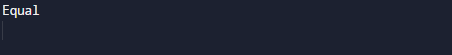For not Equal.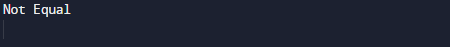In the above program, we have first declared and initialized a set variables required in the program.

• strng1 = it will hold first String value.
• strng2 = it will hold Second String value.

And  in the next statement we will compare these two string and if they are equal we print Equal in output and if they  are not equal we will print they are not  equal let have a look at the images below.

First we declare string first and second same as you can see in image below.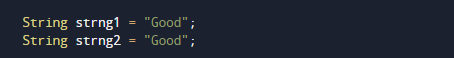then we will check condition in if else statement that if string are equal then we will print equalthen in the next method we will add two different strings and then compare them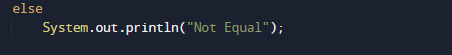in that condition  if else returns false value then we will jump to else condition where we will print string are not equal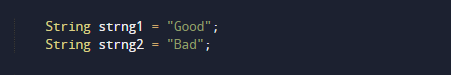As shown in the image above that string are not equal so in this program we check that the given string in variable one and two are equal or not.

In this tutorial we have learn about the Java Program to Compare Two Strings and its application with practical example. I hope you will like this tutorial.